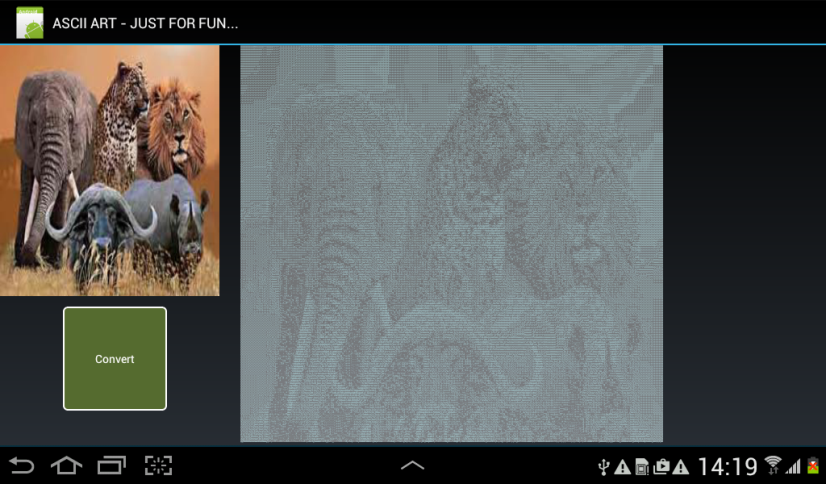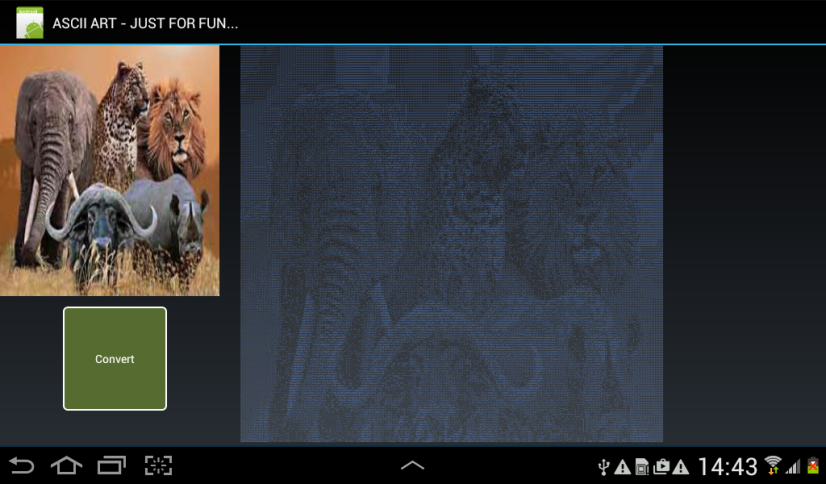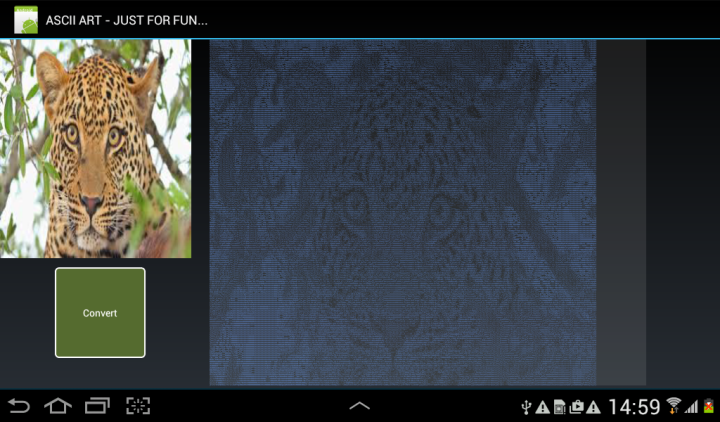# Android ExampleASCII ART

Have done this purely for the fun of it. The project uses 70 ASCII characters to generate an ASCII ART image from the bitmap. The ASCII ART image is actually a label with the label's text set to the ASCII string that was generated from the bitmap (image on the left). Have used a text size of 2 for the label and have set the typeface to MONOSPACE. Note that the project uses inline Java code and you will need a B4A version that supports inline Java code to run the project (with the JavaObject library enabled).

This is a very oversimplified way of doing it - have just used the average of each pixel's RGB values to decide upon the ASCII character to be used for each pixel. Thus, every pixel in the bitmap is represented by an ASCII character in the ASCII ART image.

I have done this on a 7" tablet so that the display can fit the ASCII image. The image can be reduced if one for eg take blocks of let's say 5 x 5 pixels and work out what character would best represent the 5 x 5 pixels. It can get quite scientific and will involve far more math calcs than the simple approach that I have followed here. But nevertheless, it was an interesting exercise...

The ASCII ART image is not wonderfully clear but at least one can catch the drift. By following other techniques some wonderful results can be obtained.#### Attachments

• b4aAsciiArt.zip
21.2 KB · Views: 382
Last edited:

#### Johan Schoeman

##### Expert
Longtime User
Replace this code in the inline Java code....
B4X:
``````if (x == bmp.getWidth() - 1)
html.append("&lt;br/&rt");

....with this.....

if (x == bmp.getWidth() - 1)
html.append((char) 10);``````

....and just make sure that the label is wide enough (rather too wide than too narrow). html.append((char) 10) forces a CRLF at the end of every line that was scanned across the width of the bitmap. The code as it was did not force the required CRLF's in the text of the label.

Also, change the text color of the label if you want the ASCII ART pic to be a different color

Last edited:

#### Johan Schoeman

##### Expert
Longtime User
Replace the whole inline Java code method called
private static String getGrayShade(float redValue) {
....
.....
....
}

....with the below code. It will eliminate the "picture negative" effect that you see. Light colors in the bitmap will now be light and dark colors in the bitmap will now be dark in the ASCII ART image....

B4X:
``````private static  String getGrayShade(float redValue) {

String asciival = " ";

if (redValue >= 69* 256/70)
{
asciival = TEN;
}
else if (redValue >= 68* 256/70)
{
asciival = NINE;
}
else if (redValue >= 67* 256/70)
{
asciival = EIGHT;
}
else if (redValue >= 66* 256/70)
{
asciival = SEVEN;
}
else if (redValue >= 65* 256/70)
{
asciival = SIX;
}
else if (redValue >= 64* 256/70)
{
asciival = FIVE;
}
else if (redValue >= 63* 256/70)
{
asciival = FOUR;
}
else if (redValue >= 62* 256/70)
{
asciival = THREE;
}
else if (redValue >= 61* 256/70)
{
asciival = TWO;
}
else if (redValue >= 60* 256/70)
{
asciival = ONE;
}
else if (redValue >= 59* 256/70)
{
asciival = TEN1;
}
else if (redValue >= 58* 256/70)
{
asciival = NINE1;
}
else if (redValue >= 57* 256/70)
{
asciival = EIGHT1;
}
else if (redValue >= 56* 256/70)
{
asciival = SEVEN1;
}
else if (redValue >= 55* 256/70)
{
asciival = SIX1;
}
else if (redValue >= 54* 256/70)
{
asciival = FIVE1;
}
else if (redValue >= 53* 256/70)
{
asciival = FOUR1;
}
else if (redValue >= 52* 256/70)
{
asciival = THREE1;
}
else if (redValue >= 51* 256/70)
{
asciival = TWO1;
}
else if (redValue >= 50* 256/70)
{
asciival = ONE1;
}
else if (redValue >= 49* 256/70)
{
asciival = TEN2;
}
else if (redValue >= 48* 256/70)
{
asciival = NINE2;
}
else if (redValue >= 47* 256/70)
{
asciival = EIGHT2;
}
else if (redValue >= 46* 256/70)
{
asciival = SEVEN2;
}
else if (redValue >= 45* 256/70)
{
asciival = SIX2;
}
else if (redValue >= 44* 256/70)
{
asciival = FIVE2;
}
else if (redValue >= 43* 256/70)
{
asciival = FOUR2;
}
else if (redValue >= 42* 256/70)
{
asciival = THREE2;
}
else if (redValue >= 41* 256/70)
{
asciival = TWO2;
}
else if (redValue >= 40* 256/70)
{
asciival = ONE2;
}
else if (redValue >= 39* 256/70)
{
asciival = TEN3;
}
else if (redValue >= 38* 256/70)
{
asciival = NINE3;
}
else if (redValue >= 37* 256/70)
{
asciival = EIGHT3;
}
else if (redValue >= 36* 256/70)
{
asciival = SEVEN3;
}
else if (redValue >= 35* 256/70)
{
asciival = SIX3;
}
else if (redValue >= 34* 256/70)
{
asciival = FIVE3;
}
else if (redValue >= 33* 256/70)
{
asciival = FOUR3;
}
else if (redValue >= 32* 256/70)
{
asciival = THREE3;
}
else if (redValue >= 31* 256/70)
{
asciival = TWO3;
}
else if (redValue >= 30* 256/70)
{
asciival = ONE3;
}
else if (redValue >= 29* 256/70)
{
asciival = TEN4;
}
else if (redValue >= 28* 256/70)
{
asciival = NINE4;
}
else if (redValue >= 27* 256/70)
{
asciival = EIGHT4;
}
else if (redValue >= 26* 256/70)
{
asciival = SEVEN4;
}
else if (redValue >= 25* 256/70)
{
asciival = SIX4;
}
else if (redValue >= 24* 256/70)
{
asciival = FIVE4;
}
else if (redValue >= 23* 256/70)
{
asciival = FOUR4;
}
else if (redValue >= 22* 256/70)
{
asciival = THREE4;
}
else if (redValue >= 21* 256/70)
{
asciival = TWO4;
}
else if (redValue >= 20* 256/70)
{
asciival = ONE4;
}
else if (redValue >= 19* 256/70)
{
asciival = TEN5;
}
else if (redValue >= 18* 256/70)
{
asciival = NINE5;
}
else if (redValue >= 17* 256/70)
{
asciival = EIGHT5;
}
else if (redValue >= 16* 256/70)
{
asciival = SEVEN5;
}
else if (redValue >= 15* 256/70)
{
asciival = SIX5;
}
else if (redValue >= 14* 256/70)
{
asciival = FIVE5;
}
else if (redValue >= 13* 256/70)
{
asciival = FOUR5;
}
else if (redValue >= 12* 256/70)
{
asciival = THREE5;
}
else if (redValue >= 11* 256/70)
{
asciival = TWO5;
}
else if (redValue >= 10* 256/70)
{
asciival = ONE5;
}
else if (redValue >= 9* 256/70)
{
asciival = TEN6;
}
else if (redValue >= 8* 256/70)
{
asciival = NINE6;
}
else if (redValue >= 7* 256/70)
{
asciival = EIGHT6;
}
else if (redValue >= 6* 256/70)
{
asciival = SEVEN6;
}
else if (redValue >= 5* 256/70)
{
asciival = SIX6;
}
else if (redValue >= 4* 256/70)
{
asciival = FIVE6;
}
else if (redValue >= 3* 256/70)
{
asciival = FOUR6;
}
else if (redValue >= 2* 256/70)
{
asciival = THREE6;
}
else if (redValue >= 1* 256/70)
{
asciival = TWO6;
}
else
{
asciival = ONE6;
}
return asciival;
}``````

#### DonManfred

##### Expert
Longtime User
The project uses 70 ASCII characters to generate an ASCII ART image
Nice one! In the past i used a lot of ASCII-Art...

See this which we have done in the mid 90sPer default the "Muhwie" (we called them like this) No 49 is generated into an animated gif and then showed the gif. attached you find php-Script and the "Muhwie-Files" we created.

You can find them online here. My answer to Holgers Train-Muhwie was this one (attention: rated age 18+)#### Attachments

• muhwies.zip
229.2 KB · Views: 298
Last edited:

#### stevel05

##### Expert
Longtime User
Taking it to the extreme : http://www.asciimation.co.nz/, Some people have too much time on their hands.•Johan Schoeman and DonManfred

#### Johan Schoeman

##### Expert
Longtime User
.....sorry, really stuffing around here but better that I post an updated project. Have changed the text color, incorporated the change in post 3 above, and have set the panel background color to WHITE with an alpha of 100. See pic below. Amazing, all done with text....And with the panel background alpha set to 25....#### Attachments

• b4aAsciiArt.zip
68.5 KB · Views: 319
Last edited:
•stevel05 and DonManfred

#### sorex

##### Expert
Longtime User
Well done, Johan.

This reminds me of these pages that were passing by in the 80s printed with old (new at that time) 9 or 24 pin matrix printers and representing a jesus or naked ladyyou should check out ANSI (BBS gfx) & PETSCII (C64) art aswell where colors are used aswell.
an ANSI convertor would be even easier than this while PETSCII is really tricky.

•Johan Schoeman

#### Daniel-White

##### Active Member
Longtime User
I did a test in Andy emulator and work nice. Only one question who isLena•Johan Schoeman

#### Johan Schoeman

##### Expert
Longtime User
I did a test in Andy emulator and work nice. Only one question who isLenaYou can Google her Daniel....and only dream....She goes like GGGRRRR..........Last edited:
•Daniel-White

Replies
0
Views
1K
Android Question Write ASCII characters
Replies
8
Views
1K
Replies
0
Views
2K
B4A Library ASCII-Art-Generator
Replies
0
Views
2K
Replies
6
Views
2K